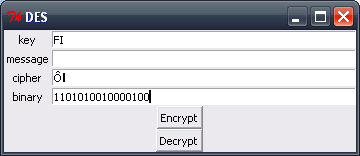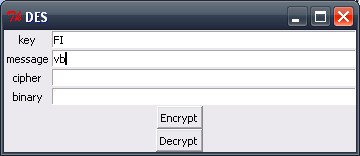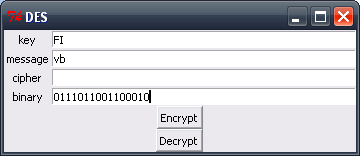Lennu.net

# Data Encryption Standard Made With Key Sizes of 14 BitsToday I would like to share you the power of cryptography.

I made this software 2011 in Eindhoven, Netherlands (country of cryptography) where I was having my exchange studies at the time.

The software is about the Data Encryption Standard or DES, however you like to call it.

## DES With 14 Bits #

Data Encryption Standard is the old queen of cryptography, which eventually got caught for being too easy to solve. There is a great article in Wikipedia about the topic and how it has influenced modern cryptography.

What I did was kinda experiment if I could code this thing together with some tweaking to the original Standard. DES was created to encrypt with key sizes of 56 bits and block sizes of 64 bits doing 16 rounds of crypting in total.

To learn the standard and the basics of cryptography, I chose to do it with key sizes of 14 bits and block sizes of 16 bits doing 2 rounds of crypting in total. I actually succeeded on this and did a little software with Python to prove it.

## Mathematics #

Here are the mathematics from my version of the Data Encryption Standard.

### Permutations #

``````PC1 = [12,  5, 14,  1, 10,  2, 6,
9, 15,  4, 13,  7, 11, 3]

PC2 = [  6, 11, 4,  8, 13, 3,
12,  5, 1, 10,  2, 9]

IP = [   2, 14,  6, 10,
12,  8, 16,  4,
5, 13,  3,  9,
11,  1, 15,  7]

IP-1 = [ 14, 1, 11, 8,
9, 3, 16, 6,
12, 4, 13, 5,
10, 2, 15, 7]

E = [   8, 1, 2, 3, 4, 5,
4, 5, 6, 7, 8, 1]

S = [
[ 4, 11,  2, 14, 15, 0,  8, 13,  3, 12, 9,  7,  5, 10, 6,  1,
13,  0, 11,  7,  4, 9,  1, 10, 14,  3, 5, 12,  2, 15, 8,  6,
1,  4, 11, 13, 12, 3,  7, 14, 10, 15, 6,  8,  0,  5, 9,  2,
6, 11, 13,  8,  1, 4, 10,  7,  9,  5, 0, 15, 14,  2, 3, 12],

[13,  2,  8, 4,  6, 15, 11,  1, 10,  9,  3, 14,  5,  0, 12,  7,
1, 15, 13, 8, 10,  3,  7,  4, 12,  5,  6, 11,  0, 14,  9,  2,
7, 11,  4, 1,  9, 12, 14,  2,  0,  6, 10, 13, 15,  3,  5,  8,
2,  1, 14, 7,  4, 10,  8, 13, 15, 12,  9,  0,  3,  5,  6, 11]
]

P = [   6, 4, 7, 3,
5, 1, 8, 2]
``````

### Calculations #

We choose a sub key K’ of 12 bits

``````000110 110000
``````

Calculations of F(R, K’) as R = 1011 0110

``````E(R) = 010110 101101
K (+) E(R) = 010000 011101
S7(B1)S8(B2) = 0011 1001
F(R, K') = 0101 1010
``````

We choose a key K of 16 bits

``````0100 0110 0100 1001
``````

And calculate the two keys K1 and K2

``````K+ = 0000 1110 0011 00
C0 = 0000111
D0 = 0001100
C1 = 0111000
D1 = 1100000
C2 = 1000011
D2 = 0000110
K1 = 001101000011
K2 = 100010101000
``````

We choose an input I of 15 bits (e.g. the ASCI-coding of the characters ‘v’ and ‘b’)

``````0111 0110 0110 0001
``````

And encrypt I using the DESHI-algorithm where we use the given IP and the calculated K1, K2 and IP-1.

``````IP = 1011 0001 0010 1011
L0 = 1011 0001
R0 = 0010 1011
R1 = L0 (+) F(R0, K1)
E(R0) = 100101 010110
K1 = 001101000011
K1 (+) E(R0) = 101000 010101
S7(B1) S8(B2) = 1100 0110
F(R, K’) = 1010 0101
R1 = L0 (+) F(R0, K1) = 0001 0100
L1 = R0 = 0010 1011
R2 = L0 (+) F(R1, K2)
E(R1) = 000010 101000
K2 = 1000010 101000
K2 (+) E(R1) = 100000 000000
S7(B1) S8(B2) = 0001 1101
F(R, K’) = 1100 1010
R2 = L1 (+) F(R1, K2) = 1110 0001
L2 = R0 = 0001 0100
R2L2 = 1110 0001 0001 0100

IP-1 = 1101 0100 1000 0100
``````

## Software #

Program is made with Python version 3.2. The program can probably be run if the system has 3.0 or newer version of Python, but only tested with 3.2. The latest version can be downloaded from: http://www.python.org/download/. The program can be used in Linux or Windows as long as there is Python installed into the system.

Here are some screenshots with the same examples as used in the earlier handwritten DES encryption.After clicking the encrypt button.After clicking the decrypt button### Usage #

This was just an experiment if I could learn DES and some cryptography, this software should not be used in real life situations. The method I used actually made DES weaker against attacks because I did it with less bits than the usual version. I hope maybe someone may find this information useful and maybe learn some cryptography. In my opinion it’s a really complex world and so much different from traditional software development, because of the mathematics that are included.

### Source Code #

Here is the source code for this software. To run the software, just copy/paste the code into .py file and run it.

``# GNU GPL# des.py - Python version used: 3.2 from tkinter import *	# UI tool importfrom tkinter.messagebox import showerror	# copies showerrorfieldnames = ('key', 'message', 'cipher', 'binary') pc1 = [12,  5, 14,  1, 10,  2, 6,	9, 15,  4, 13,  7, 11, 3] leftshifts = [3, 3] pc2 = [  6, 11, 4,  8, 13, 3,	12,  5, 1, 10,  2, 9] ip = [   2, 14,  6, 10,	12,  8, 16,  4,	 5, 13,  3,  9,	11,  1, 15,  7] e = [   8, 1, 2, 3, 4, 5,	4, 5, 6, 7, 8, 1]s = [	[ 4, 11,  2, 14, 15, 0,  8, 13,  3, 12, 9,  7,  5, 10, 6,  1,	 13,  0, 11,  7,  4, 9,  1, 10, 14,  3, 5, 12,  2, 15, 8,  6,	  1,  4, 11, 13, 12, 3,  7, 14, 10, 15, 6,  8,  0,  5, 9,  2,	  6, 11, 13,  8,  1, 4, 10,  7,  9,  5, 0, 15, 14,  2, 3, 12], 	[13,  2,  8, 4,  6, 15, 11,  1, 10,  9,  3, 14,  5,  0, 12,  7,	  1, 15, 13, 8, 10,  3,  7,  4, 12,  5,  6, 11,  0, 14,  9,  2,	  7, 11,  4, 1,  9, 12, 14,  2,  0,  6, 10, 13, 15,  3,  5,  8,	  2,  1, 14, 7,  4, 10,  8, 13, 15, 12,  9,  0,  3,  5,  6, 11]]p = [   6, 4, 7, 3,	5, 1, 8, 2] ip1 = [ 14, 1, 11, 8,	 9, 3, 16, 6,	12, 4, 13, 5,	10, 2, 15, 7] def makeWidgets():	# makeWidgets function	global entries	window = Tk()	window.title('DES')	form = Frame(window)	form.pack()	entries = {}	for(ix, label) in enumerate(fieldnames):	# creation of labels and entries into two columns		lab = Label(form, text=label)		ent = Entry(form, width=50)		lab.grid(row=ix, column=0)		ent.grid(row=ix, column=1)		entries[label] = ent	Button(window, text='Encrypt', command=en).pack(side=TOP)	# encrypt button, click takes to function en()	Button(window, text='Decrypt', command=de).pack(side=TOP)	# decrypt button, click takes to function de()	return window def stringToBits(data):	# string to bits function	data = [ord(c) for c in data]	# creates unicodes to list	result = []	for ch in data:		i = 7		while i >= 0:	# bit selection			if ch & (1 << i) != 0:				result.append(1)			else:				result.append(0)			i -= 1	return result	# returns a list of created bits def bitsToString(bits):	# bits to string function	strinG = []	position = 0	a = 0	while position < len(bits):		a += bits[position] << (7 - (position % 8))		if (position % 8) == 7:			strinG.append(a)			a = 0		position += 1	return ''.join([chr(a) for a in strinG])	# returns a string of characters made from bytes def permutate(table, block):	# permutation function	return list(map(lambda x: block[x], table))	# returns the permutated list def createSubkeys(key, pc1, pc2, leftshifts):	# subkeys creation	key = permutate(pc1,  + stringToBits(key))	# pc1 permutation	i = 0	L = key[:7]	# slices the permutation into LEFT and RIGHT	R = key[7:]	subkeys = []	while i < 2:		j = 0		while j < leftshifts[i]: # leftshifts the bits from beginning to end			L.append(L)			del L			R.append(R)			del R			j += 1		subkeys.append(permutate(pc2,  + L + R))	# pc2 permutation		i += 1	return subkeys def des(message, ip, e, s, p, ip1, subkeys, crypt):	# des function	if not message:		return ""	if len(message) % 2 != 0:		showerror(title='Error', message='The message or encrypted data must be a multiple of 16 bits!')		return ""	a = 0	result = []	while a < len(message):		cryptob = stringToBits(message[a:a+2])	# gets part of the string and turns it to bits		ipresult = permutate(ip,  + cryptob)	# ip permutation		L = ipresult[:8]	# slices the variable into LEFT and RIGHT		R = ipresult[8:]		if crypt == 'en':	# sets the variables for the encryption or decryption			it = 0			itadd = 1		if crypt == 'de':			it = 1			itadd = -1		i = 0		while i < 2:	# encryption			R2 = R[:]			R = permutate(e,  + R)	# E permutation			R = list(map(lambda x, y: x ^ y, R, subkeys[it])) # XOR the permutation			B = [R[:6], R[6:]]	# slices the permutation into list B and B			k = 0			bg =  * 8			position = 0			while k < 2:				f = (B[k] << 1) + B[k]	# for clarification << means left shifting and >> right shifting				g = (B[k] << 3) + (B[k] << 2) + (B[k] << 1) + B[k]				h = s[k][(f << 4) + g] 				bg[position] = (h & 8) >> 3				bg[position + 1] = (h & 4) >> 2				bg[position + 2] = (h & 2) >> 1				bg[position + 3] = h & 1				position += 4				k += 1			R = permutate(p,  + bg)	# P permutation			R = list(map(lambda x, y: x ^ y, R, L))	# XOR the permutation			L = R2			i += 1			it += itadd		ip1result = permutate(ip1,  + R + L)	# ip-1 permutation		result.append(bitsToString(ip1result))	# adds the crypted part as string to result		a += 2	return ''.join(result)	# returns result list as one joined string def en():	#encrypt click	crypt_click('en') def de(): 	#decrypt click	crypt_click('de') def crypt_click(crypttype):	#process of encryption or decryption depending of the click	key = entries['key'].get()	# gets the key from the UI	if not key:		showerror(title='Error', message='You have to enter a key!')	else:		if len(key) % 2 != 0:			showerror(title='Error', message='The key must be 16 bits!')		else:			subkeys = createSubkeys(key, pc1, pc2, leftshifts)	# creation of subkeys in createSubkeys() functions			if crypttype == 'en':	# sets the variables to message or to cipher depending on what the user clicked				textfrom = 'message'				textto = 'cipher'				entries['cipher'].delete(0, END)			else:				textfrom = 'cipher'				textto = 'message'				messageOrCipher = entries[textfrom].get()	# gets message or cipher			result = des(messageOrCipher, ip, e, s, p, ip1, subkeys, crypttype)	# actual result from the des() function			binary = ''.join(map(str, stringToBits(result)))	# makes the binary of the result			entries[textfrom].delete(0, END)	# UI entry delete			entries[textto].insert(0, result)	# UI entry insert			entries['binary'].delete(0, END)			entries['binary'].insert(0, binary)			 window = makeWidgets()	# creates the UIwindow.mainloop()	# mainloop of the UI to get actions``